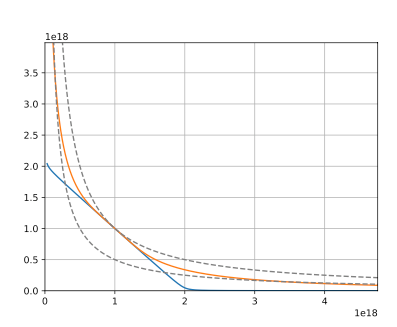# Pricing Algorithm

Danaswap’s pricing algorithm for trades is based on invariant equations. For each contract there is an equation which must always hold true (the invariant equation), and by solving this equation after adjusting some variables, we get the price at which a given trade would be executed at a given point in time.Danaswap's invariant equation• Dashed lines: Constant product invariants
• Blue line: Stable-Pool invariant
• Orange line: Extended Stable-Pool invariant
Essentially, the invariant equation represents how the exchange rate between two assets changes given supply and demand. In other words, if the supply of an asset i in the liquidity pool declines relative to other assets in the pool, then the relative price of i increases, and vice versa.

## Optimal Pool Proportion

Individual assets within a StablePool each consume a proportion of the whole pool. The desirable proportion is called the Optimal Pool Proportion (OPP). When a user swaps using an unbalanced StablePool, or causes it to be unbalanced by swapping, the effect of their swap on the OPP will result in a rebalancing fee or bonus being applied to the user. This adjustment is applied by increasing/reducing the quantity of output tokens for the swap in accordance with its effect on the StablePool’s OPP.
• For swaps:
• Rebalancing Fees are charged by deducting the output of any swap that will increase the deviation from the OPP within a StablePool.
• Rebalancing Bonuses are added to the output of any swap that will reduce the deviation from the OPP within a StablePool.
• For liquidity providers:
• Rebalancing Fees are charged by reducing the amount of received Liquidity Pool (LP) tokens for any LP deposit which increases a StablePool’s deviation from its OPP.
• Rebalancing Bonuses are paid by increasing the amount of received LP tokens for any LP deposit that reduces a StablePool’s deviation from its OPP.
The calculation of a swap’s fee or bonus is based on the magnitude of the effect that swap has on the deviation from the OPP. The more unbalanced a given pool is, the higher the Rebalancing Fee will be for a swap causing it to further deviate from the OPP, and conversely the higher the Rebalancing Bonus will be for those performing swaps which cause the pool to converge more to its OPP.